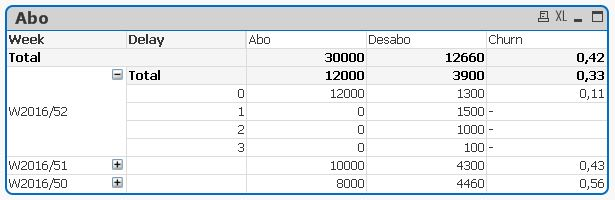# QlikView App Development

Discussion Board for collaboration related to QlikView App Development.

Highlighted
Contributor

## Churn Calculation in crosstab

Hello,

I have a table with : Week, delay, subs, unsubs.

I want to calculate the churn (unsubs/subs) by week and delay.

If I use "sum(unsubs)/sum(subs)", the formula works when the table is collapsed.But If I expend the table, the formula no longer works because subs take place on delay 0 and unsubs may occur in other delay.So I use this formula:

if(Dimensionality()=0 or IsNull(rowno()) or rowno()=0 or (Dimensionality()=1 and rowno()>0)

,sum(Desabo)/sum(Abo)

,sum(Desabo)/rangesum(above(sum(Abo),0,rowno()))

)

But I'd like to find a formula which don't need to use the condition "if(Dimensionality()=0 or IsNull(rowno()) or rowno()=0 or (Dimensionality()=1 and rowno()>0)".

Is there another way to do this?

Sara

Tags (2)
1 Solution

Accepted SolutionsMVP

## Re: Churn Calculation in crosstab

May be this:

Sum(Desabo)/Sum(TOTAL <Week>Abo)